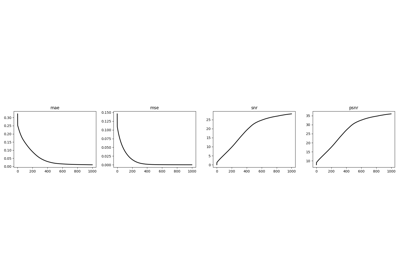# pylops.optimization.cls_sparsity.ISTA#

class pylops.optimization.cls_sparsity.ISTA(Op, callbacks=None)[source]#

Iterative Shrinkage-Thresholding Algorithm (ISTA).

Solve an optimization problem with $$L_p, \; p=0, 0.5, 1$$ regularization, given the operator Op and data y. The operator can be real or complex, and should ideally be either square $$N=M$$ or underdetermined $$N<M$$.

Parameters
Oppylops.LinearOperator

Operator to invert

Raises
NotImplementedError

If threshkind is different from hard, soft, half, soft-percentile, or half-percentile

ValueError

If perc=None when threshkind is soft-percentile or half-percentile

ValueError

If monitorres=True and residual increases

OMP

Orthogonal Matching Pursuit (OMP).

FISTA

Fast Iterative Shrinkage-Thresholding Algorithm (FISTA).

SPGL1

Spectral Projected-Gradient for L1 norm (SPGL1).

SplitBregman

Split Bregman for mixed L2-L1 norms.

Notes

Solves the following synthesis problem for the operator $$\mathbf{Op}$$ and the data $$\mathbf{y}$$:

$J = \|\mathbf{y} - \mathbf{Op}\,\mathbf{x}\|_2^2 + \epsilon \|\mathbf{x}\|_p$

or the analysis problem:

$J = \|\mathbf{y} - \mathbf{Op}\,\mathbf{x}\|_2^2 + \epsilon \|\mathbf{SOp}^H\,\mathbf{x}\|_p$

if SOp is provided. Note that in the first case, SOp should be assimilated in the modelling operator (i.e., Op=GOp * SOp).

The Iterative Shrinkage-Thresholding Algorithms (ISTA)  is used, where $$p=0, 0.5, 1$$. This is a very simple iterative algorithm which applies the following step:

$\mathbf{x}^{(i+1)} = T_{(\epsilon \alpha /2, p)} \left(\mathbf{x}^{(i)} + \alpha\,\mathbf{Op}^H \left(\mathbf{y} - \mathbf{Op}\,\mathbf{x}^{(i)}\right)\right)$

or

$\mathbf{x}^{(i+1)} = \mathbf{SOp}\,\left\{T_{(\epsilon \alpha /2, p)} \mathbf{SOp}^H\,\left(\mathbf{x}^{(i)} + \alpha\,\mathbf{Op}^H \left(\mathbf{y} - \mathbf{Op} \,\mathbf{x}^{(i)}\right)\right)\right\}$

where $$\epsilon \alpha /2$$ is the threshold and $$T_{(\tau, p)}$$ is the thresholding rule. The most common variant of ISTA uses the so-called soft-thresholding rule $$T(\tau, p=1)$$. Alternatively an hard-thresholding rule is used in the case of $$p=0$$ or a half-thresholding rule is used in the case of $$p=1/2$$. Finally, percentile bases thresholds are also implemented: the damping factor is not used anymore an the threshold changes at every iteration based on the computed percentile.

1

Daubechies, I., Defrise, M., and De Mol, C., “An iterative thresholding algorithm for linear inverse problems with a sparsity constraint”, Communications on pure and applied mathematics, vol. 57, pp. 1413-1457. 2004.

Methods

 __init__(Op[, callbacks]) callback(x, *args, **kwargs) Callback routine finalize([show]) Finalize solver run(x[, niter, show, itershow]) Run solver setup(y[, x0, niter, SOp, eps, alpha, ...]) Setup solver solve(y[, x0, niter, SOp, eps, alpha, ...]) Run entire solver step(x[, show]) Run one step of solver

## Examples using pylops.optimization.cls_sparsity.ISTA#